# 25 Results

View
Selected filters:
• MCCRS.Math.Content.8.G.B.7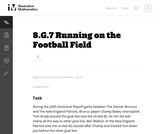Unrestricted Use
CC BY
Rating

This is a task from the Illustrative Mathematics website that is one part of a complete illustration of the standard to which it is aligned. Each task has at least one solution and some commentary that addresses important asects of the task and its potential use. Here are the first few lines of the commentary for this task: During the 2005 Divisional Playoff game between The Denver Broncos and The New England Patriots, Bronco player Champ Bailey intercepted Tom Brady aroun...

Subject:
Mathematics
Material Type:
Activity/Lab
Provider:
Illustrative Mathematics
Provider Set:
Illustrative Mathematics
Author:
Illustrative Mathematics
03/17/2013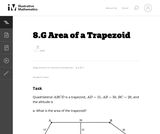Unrestricted Use
CC BY
Rating

This is a task from the Illustrative Mathematics website that is one part of a complete illustration of the standard to which it is aligned. Each task has at least one solution and some commentary that addresses important asects of the task and its potential use. Here are the first few lines of the commentary for this task: Quadrilateral $ABCD$ is a trapezoid, $AD = 15$, $AB = 50$, $BC = 20$, and the altitude is 12. What is the area of the trapezoid?...

Subject:
Mathematics
Material Type:
Activity/Lab
Provider:
Illustrative Mathematics
Provider Set:
Illustrative Mathematics
Author:
Illustrative Mathematics
03/17/2013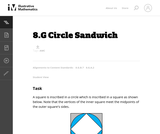Unrestricted Use
CC BY
Rating

This is a task from the Illustrative Mathematics website that is one part of a complete illustration of the standard to which it is aligned. Each task has at least one solution and some commentary that addresses important asects of the task and its potential use. Here are the first few lines of the commentary for this task: A square is inscribed in a circle which is inscribed in a square as shown below. Note that the vertices of the inner square meet the midpoints of the o...

Subject:
Mathematics
Material Type:
Activity/Lab
Provider:
Illustrative Mathematics
Provider Set:
Illustrative Mathematics
Author:
Illustrative Mathematics
09/08/2013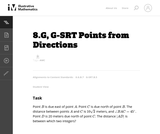Unrestricted Use
CC BY
Rating

This is a task from the Illustrative Mathematics website that is one part of a complete illustration of the standard to which it is aligned. Each task has at least one solution and some commentary that addresses important asects of the task and its potential use. Here are the first few lines of the commentary for this task: Point $B$ is due east of point $A$. Point $C$ is due north of point $B$. The distance between points $A$ and $C$ is $10\sqrt 2$ meters, and \angle BAC... Subject: Mathematics Material Type: Activity/Lab Provider: Illustrative Mathematics Provider Set: Illustrative Mathematics Author: Illustrative Mathematics Date Added: 03/29/2013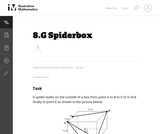Unrestricted Use CC BY Rating This is a task from the Illustrative Mathematics website that is one part of a complete illustration of the standard to which it is aligned. Each task has at least one solution and some commentary that addresses important asects of the task and its potential use. Here are the first few lines of the commentary for this task: A spider walks on the outside of a box from point A to B to C to D and finally to point E as shown in the picture below. Draw a net of the box and map ... Subject: Mathematics Material Type: Activity/Lab Provider: Illustrative Mathematics Provider Set: Illustrative Mathematics Author: Illustrative Mathematics Date Added: 11/25/2013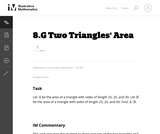Unrestricted Use CC BY Rating This is a task from the Illustrative Mathematics website that is one part of a complete illustration of the standard to which it is aligned. Each task has at least one solution and some commentary that addresses important asects of the task and its potential use. Here are the first few lines of the commentary for this task: LetA$be the area of a triangle with sides of length 25, 25, and 30. Let$B$be the area of a triangle with sides of length 25, 25, and 40. Find$A/B...

Subject:
Mathematics
Material Type:
Activity/Lab
Provider:
Illustrative Mathematics
Provider Set:
Illustrative Mathematics
Author:
Illustrative Mathematics
05/29/2013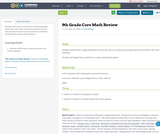Conditional Remix & Share Permitted
CC BY-NC
Rating

Students will create an interactive Powerpoint game where they will create real world problems that are used as clues to move on to the next level. Problems include all 8th grade math standards.

Subject:
Mathematics
Material Type:
Interactive
Author:
Linda Ridings
01/28/2016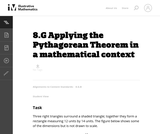Unrestricted Use
CC BY
Rating

This task requires students to apply the Pythagorean Theorem.

Subject:
Mathematics
Geometry
Material Type:
Activity/Lab
Provider:
Illustrative Mathematics
Provider Set:
Illustrative Mathematics
Author:
Illustrative Mathematics
05/01/2012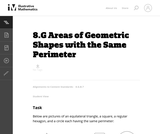Unrestricted Use
CC BY
Rating

This problem is part of a very rich tradition of problems looking to maximize the area enclosed by a shape with fixed perimeter. Only three shapes are considered here because the problem is difficult for more irregular shapes.

Subject:
Mathematics
Geometry
Material Type:
Activity/Lab
Provider:
Illustrative Mathematics
Provider Set:
Illustrative Mathematics
Author:
Illustrative Mathematics
01/20/2013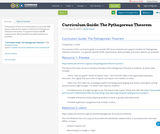Conditional Remix & Share Permitted
CC BY-NC
Rating

The purpose of this curriculum guide is to provide OER lesson material and support activities for Pythagorean Theorem instruction. It is geared towards GED® requirements. Both printable and online options are provided.

Subject:
Mathematics
Material Type:
Activity/Lab
Assessment
Lesson
Teaching/Learning Strategy
Author:
Shana Friend
05/30/2018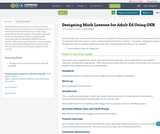Conditional Remix & Share Permitted
CC BY-NC-SA
Rating

Adult Educators do not have a lot of time to dive into dozens of resources spread all over the internet to try to find the essential items they need to create a high-quality lesson for their students. This guide is designed to walk you through each step of creating a math lesson-plan with recommended OER that you can evaluate.

With each step of the guide, I create a sample lesson on learning the Pythagorean Theorem. By the end, you have an entire lesson plan example for this concept.

Subject:
Mathematics
Material Type:
Activity/Lab
Lesson Plan
Teaching/Learning Strategy
Author:
Jason Walker
05/18/2018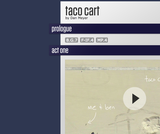Conditional Remix & Share Permitted
CC BY-NC
Rating

The 3 act math is a perfect way to incorporate the See-Think-Wonder activity.  Dan Meyer is the originator of the 3 act math plays and the Pearson Envisions Math textbook incorporates one every Topic/Chapter.  I am writing this lesson plan with the idea of using the Taco Cart 3 act math play.The link to the 3-act-math play is below.Taco Cart http://threeacts.mrmeyer.com/tacocart/

Subject:
Mathematics
Material Type:
Lesson Plan
Author:
Jill Oliver
11/05/2018Educational Use
Rating

Students use simple materials to design an open spectrograph so they can calculate the angle light is bent when it passes through a holographic diffraction grating. A holographic diffraction grating acts like a prism, showing the visual components of light. After finding the desired angles, students use what they have learned to design their own spectrograph enclosure.

Subject:
Engineering
Physics
Material Type:
Activity/Lab
Provider:
TeachEngineering
Provider Set:
TeachEngineering
10/14/2015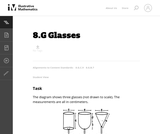Unrestricted Use
CC BY
Rating

This task gives students an opportunity to work with volumes of cylinders, spheres and cones. Notice that the insight required increases as you move across the three glasses, from a simple application of the formula for the volume of a cylinder, to a situation requiring decomposition of the volume into two pieces, to one where a height must be calculated using the Pythagorean theorem.

Subject:
Mathematics
Geometry
Material Type:
Activity/Lab
Provider:
Illustrative Mathematics
Provider Set:
Illustrative Mathematics
Author:
Illustrative Mathematics
05/01/2012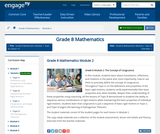Conditional Remix & Share Permitted
CC BY-NC-SA
Rating

In this module, students learn about translations, reflections, and rotations in the plane and, more importantly, how to use them to precisely define the concept of congruence. Throughout Topic A, on the definitions and properties of the basic rigid motions, students verify experimentally their basic properties and, when feasible, deepen their understanding of these properties using reasoning. All the lessons of Topic B demonstrate to students the ability to sequence various combinations of rigid motions while maintaining the basic properties of individual rigid motions. Students learn that congruence is just a sequence of basic rigid motions in Topic C, and Topic D begins the learning of Pythagorean Theorem.

Subject:
Geometry
Material Type:
Module
Provider:
New York State Education Department
Provider Set:
EngageNY
09/21/2013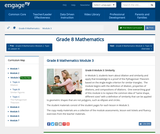Conditional Remix & Share Permitted
CC BY-NC-SA
Rating

In Module 3, students learn about dilation and similarity and apply that knowledge to a proof of the Pythagorean Theorem based on the Angle-Angle criterion for similar triangles.  The module begins with the definition of dilation, properties of dilations, and compositions of dilations.  One overarching goal of this module is to replace the common idea of same shape, different sizes with a definition of similarity that can be applied to geometric shapes that are not polygons, such as ellipses and circles.

Subject:
Geometry
Material Type:
Module
Provider:
New York State Education Department
Provider Set:
EngageNY
10/17/2013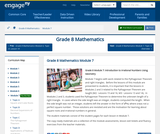Conditional Remix & Share Permitted
CC BY-NC-SA
Rating

Module 7 begins with work related to the Pythagorean Theorem and right triangles.  Before the lessons of this module are presented to students, it is important that the lessons in Modules 2 and 3 related to the Pythagorean Theorem are taught (M2:  Lessons 15 and 16, M3:  Lessons 13 and 14).  In Modules 2 and 3, students used the Pythagorean Theorem to determine the unknown length of a right triangle.  In cases where the side length was an integer, students computed the length.  When the side length was not an integer, students left the answer in the form of x2=c, where c was not a perfect square number.  Those solutions are revisited and are the motivation for learning about square roots and irrational numbers in general.

Subject:
Geometry
Material Type:
Module
Provider:
New York State Education Department
Provider Set:
EngageNY
02/02/2014Only Sharing Permitted
CC BY-NC-ND
Rating

This lesson unit is intended to help you assess how students reason about geometry and, in particular, how well they are able to: use facts about the angle sum and exterior angles of triangles to calculate missing angles; apply angle theorems to parallel lines cut by a transversal; interpret geometrical diagrams using mathematical properties to identify similarity of triangles.

Subject:
Geometry
Material Type:
Assessment
Lesson Plan
Provider:
Shell Center for Mathematical Education
U.C. Berkeley
Provider Set:
Mathematics Assessment Project (MAP)
04/26/2013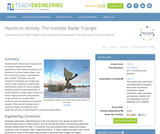Educational Use
Rating

Students learn about radar imaging and its various military and civilian applications that include recognition and detection of human-made targets, and the monitoring of space, deforestation and oil spills. They learn how the concepts of similarity and scaling are used in radar imaging to create three-dimensional models of various targets. Students apply the critical attributes of similar figures to create scale models of a radar imaging scenario using infrared range sensors (to emulate radar functions) and toy airplanes (to emulate targets). They use technology tools to measure angles and distances, and relate the concept of similar figures to real-world applications.

Subject:
Education
Material Type:
Activity/Lab
Provider:
TeachEngineering
Provider Set:
TeachEngineering
Author:
Mounir Ben Ghalia
Rocio Denise Nava
10/14/2015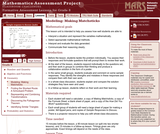Only Sharing Permitted
CC BY-NC-ND
Rating

This lesson unit is intended to help you assess how well students are able to: interpret a situation and represent the variables mathematically; select appropriate mathematical methods; interpret and evaluate the data generated; and communicate their reasoning clearly.

Subject:
Mathematics
Material Type:
Assessment
Lesson Plan
Provider:
Shell Center for Mathematical Education
U.C. Berkeley
Provider Set:
Mathematics Assessment Project (MAP)